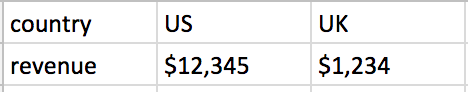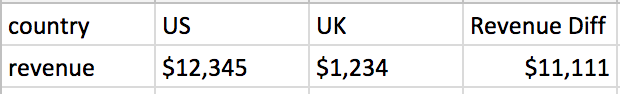# Calculate difference between two dimensions as a column?

Matt Millen shared this question ago

Is it possible to calculate the difference between a single metric across two dimensions? I.e. if we have a raw dataset that has two fields (1) country (2) revenue. If I create a tabular report with the data I would drag country dimension and revenue metric to the rows section to produce something like:Now I don't want to create a new row for differences between US and UK, but rather a new column. Simple example:Is this possible? Everything I try seems to want to create a new metric and hence a new row1

HI Matt,

The best way to do this will be to use either calculated fields or sub-queries with filters to split your revenue column, so that you can create subsequent calculated fields for the difference.

For calculated fieds you would do something like

```Calc Field: US
EQ: case when country='US' then revenue;
Calc Field: UK
EQ: case when country='UK' then revenue;```

In this case if it is not the country for that field, the value will be null and will not count when you do a sum aggregation.

If you were to use sub-queries, you would need another column to join the data on, and then add filters in each sub-query to limit the data specifically to one country.

Let me know if this makes sense.

Regards,

Nathan1

That makes sense, I was just trying to avoid going down that route when I have 20+ metrics. My actual scenario is a little more complicated as I need to compare yesterday's data against prior day, same day last week and a rolling 7 day average. And there are 29 metrics I'm comparing.

Solution I went with is to create a column for each date period (so there are 4) and then a row for each metric. It leaves me with a "metric" dimension as rows and as a result I can't format those metrics appropriately1

Hi Matt

That does sound like the better route here.